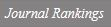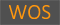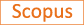Minimizing the stochasticity of halos in large-scale structure surveys

Title
Minimizing the stochasticity of halos in large-scale structure surveys
Authors
Hamaus N.Seljak U.Desjacques V.Smith R.E.Baldauf T.
Ewha Authors
Issue Date
2010
Journal Title
Physical Review D - Particles, Fields, Gravitation and Cosmology
ISSN
1550-7998Citation
Physical Review D - Particles, Fields, Gravitation and Cosmology vol. 82, no. 4
Indexed
SCIE; SCOPUSDocument Type
Article
Abstract
In recent work (Seljak, Hamaus, and Desjacques 2009) it was found that weighting central halo galaxies by halo mass can significantly suppress their stochasticity relative to the dark matter, well below the Poisson model expectation. This is useful for constraining relations between galaxies and the dark matter, such as the galaxy bias, especially in situations where sampling variance errors can be eliminated. In this paper we extend this study with the goal of finding the optimal mass-dependent halo weighting. We use N-body simulations to perform a general analysis of halo stochasticity and its dependence on halo mass. We investigate the stochasticity matrix, defined as Cij(δi-biδm)(δj-bjδm) where δm is the dark matter overdensity in Fourier space, δi the halo overdensity of the i-th halo mass bin, and bi the corresponding halo bias. In contrast to the Poisson model predictions we detect nonvanishing correlations between different mass bins. We also find the diagonal terms to be sub-Poissonian for the highest-mass halos. The diagonalization of this matrix results in one large and one low eigenvalue, with the remaining eigenvalues close to the Poisson prediction 1/n̄, where n̄ is the mean halo number density. The eigenmode with the lowest eigenvalue contains most of the information and the corresponding eigenvector provides an optimal weighting function to minimize the stochasticity between halos and dark matter. We find this optimal weighting function to match linear mass weighting at high masses, while at the low-mass end the weights approach a constant whose value depends on the low-mass cut in the halo mass function. This weighting further suppresses the stochasticity as compared to the previously explored mass weighting. Finally, we employ the halo model to derive the stochasticity matrix and the scale-dependent bias from an analytical perspective. It is remarkably successful in reproducing our numerical results and predicts that the stochasticity between halos and the dark matter can be reduced further when going to halo masses lower than we can resolve in current simulations.© 2010 The American Physical Society.
DOI
10.1103/PhysRevD.82.043515
Appears in Collections:
자연과학대학 > 물리학전공 > Journal papers
Files in This Item: